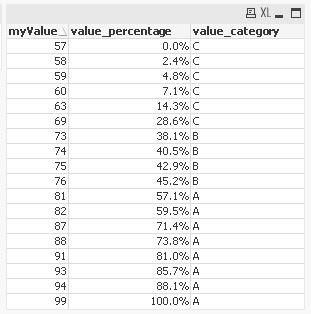# New to Qlik Sense

Discussion board where members can get started with Qlik Sense.

Announcements
Qlik® Product Spotlight: Discover what’s possible. Get more from our products.
See for yourself. Register today.
Not applicable

## Qlik sense, how to categorize a field into percentage

How to categorize a field into percentage in Qlik sense load data editor?

The column is my_value:

try to use:

(my_value - min(my_value))/(max(my_value)-min(my_value)) as value_category

and then use:

if (value_category> 0.5, 'A',

if(value_category>0.3, 'B', 'C'

))

However, the first part does not work. Any suggestion is appreciated!

1 Solution

Accepted Solutions
Not applicable

## Re: Qlik sense, how to categorize a field into percentage

Hi,

another example might be:```table1:
LOAD Ceil(Rand()*50)+50 as myValue
AutoGenerate 20;
Join
LOAD Min(myValue) as myMinValue,
Max(myValue) as myMaxValue
Resident table1;
Join
*,
If(value_percentage>0.5,'A',If(value_percentage>0.3,'B','C')) as value_category;
Num((myValue-myMinValue)/(myMaxValue-myMinValue),'0.0%') as value_percentage
Resident table1;
```

hope this helps

regards

Marco

3 Replies
Not applicable

## Re: Qlik sense, how to categorize a field into percentage

You will have to first calculate the Min and Max values of my_value. Then you can use those in the calculation afterwards.

Min(my_value) AS MinValue,

Max(my_value) AS MaxValue

FROM

[xyz.xlsx] (ooxml......);

vMinValue = Peek('MinValue');

vMaxValue = Peek('MaxValue');

(my_value - \$(vMinValue)) / ( \$(vMaxValue) - \$(vMinValue)) AS value_category,

.......

FROM

[xyz.xlsx] (ooxml......);

Not applicable

## Re: Qlik sense, how to categorize a field into percentage

Hi,

another example might be:```table1:
LOAD Ceil(Rand()*50)+50 as myValue
AutoGenerate 20;
Join
LOAD Min(myValue) as myMinValue,
Max(myValue) as myMaxValue
Resident table1;
Join
*,
If(value_percentage>0.5,'A',If(value_percentage>0.3,'B','C')) as value_category;
Num((myValue-myMinValue)/(myMaxValue-myMinValue),'0.0%') as value_percentage
Resident table1;
```

hope this helps

regards

Marco

Not applicable